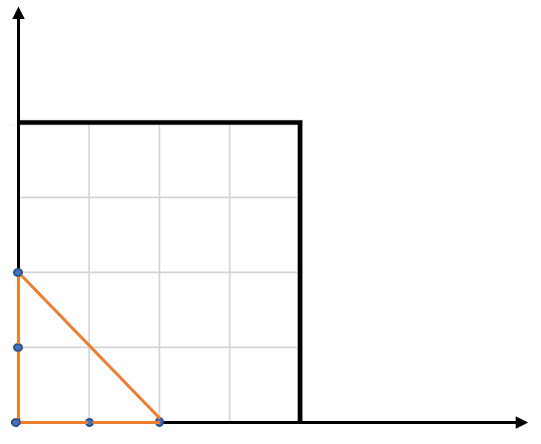LeetCode: 812. 最大三角形面积¶

1、题目描述¶• 3 <= points.length <= 50.
• 不存在重复的点。
• -50 <= points[i][j] <= 50.
• 结果误差值在 10^-6 以内都认为是正确答案。

2、解题思路¶

​ 这个题目的难点在于要知道如何利用三个点的坐标求解面积，

​ 有时间可以看看有限元分析

|x1 y1 1|
|x2 y2 1|
|x3 y3 1|
S=(1/2)*(x1y2*1+x2y3*1+x3y1*1-x1y3*1-x2y1*1-x3y2*1)

S=(1/2)*(x1y2+x2y3+x3y1-x1y3-x2y1-x3y2)
class Solution:
def largestTriangleArea(self, points):
"""
:type points: List[List[int]]
:rtype: float
"""
return max([self.getArea(point1, point2, point3) for point1, point2, point3 in itertools.combinations(points, 3)])

def getArea(self, point1, point2, point3):
(x1, y1), (x2, y2), (x3, y3) = point1, point2, point3
return 0.5 * abs(x1 * (y2 - y3) + x2 * (y3 - y1) + x3 * (y1 - y2))